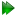Subsections

Free rotor equations

Figure 16.3: The free rotor model simulated and calculated in-frame Daeg(1) and Daeg(2) frame order matrix elements. The top row corresponds to Daeg(1) and the bottom to Daeg(2). In these plots, as no motional order parameters exist for the model, θZ corresponds to nothing. Frame order matrix values have been calculated every 10 degrees.Figure 16.4: The free rotor model simulated and calculated out-of-frame Daeg(1) and Daeg(2) frame order matrix elements. The top row corresponds to Daeg(1) and the bottom to Daeg(2). In these plots, as no motional order parameters exist for the model, θZ corresponds to nothing. Frame order matrix values have been calculated every 10 degrees.Free rotor rotation matrices

The rotation matrix is defined in equation 16.10.

Free rotor frame order matrix

The frame order matrix isThe surface normalisation factor isFree rotor 1 degree frame order

The 1 degree frame order matrix with tensor rank-2 isFree rotor 2 degree frame order

The frame order matrix in Kronecker product notation is fixed as

 Daeg(2) =. (16.20)

Free rotor frame order matrix simulation and calculation

The frame order matrix element simulation script from Section 16.2, pagewas used to compare the implementation of equations 16.19 and 16.20 above. Frame order matrix Daeg(1) and Daeg(2) values were both simulated and calculated, both within and out of the motional eigenframe. The in-frame Daeg(1) and Daeg(2) values are shown in figure 16.3. The out-of-frame Daeg(1) and Daeg(2) values are shown in figure 16.4.

The relax user manual (PDF), created 2020-08-26.